Chapter 5. Stoichiometry and the Mole

# The Mole

### Learning Objectives

1. Describe the unit mole.
2. Relate the mole quantity of substance to its mass.

So far, we have been talking about chemical substances in terms of individual atoms and molecules. Yet we don’t typically deal with substances an atom or a molecule at a time; we work with millions, billions, and trillions of atoms and molecules at a time. What we need is a way to deal with macroscopic, rather than microscopic, amounts of matter. We need a unit of amount that relates quantities of substances on a scale that we can interact with.

Chemistry uses a unit called mole. A mole (mol) is a number of things equal to the number of atoms in exactly 12 g of carbon-12. Experimental measurements have determined that this number is very large:

1 mol = 6.02214179 × 1023 things

Understand that a mole means a number of things, just like a dozen means a certain number of things—twelve, in the case of a dozen. But a mole is a much larger number of things. These things can be atoms, or molecules, or eggs; however, in chemistry, we usually use the mole to refer to the amounts of atoms or molecules. Although the number of things in a mole is known to eight decimal places, it is usually fine to use only two or three decimal places in calculations. The numerical value of things in a mole is often called Avogadro’s number (NA), which is also known as the Avogadro constant, after Amadeo Avogadro, an Italian chemist who first proposed its importance.

### Example 3

How many molecules are present in 2.76 mol of H2O? How many atoms is this?

Solution

The definition of a mole is an equality that can be used to construct a conversion factor. Also, because we know that there are three atoms in each molecule of H2O, we can also determine the number of atoms in the sample.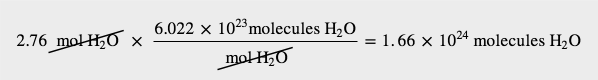To determine the total number of atoms, we have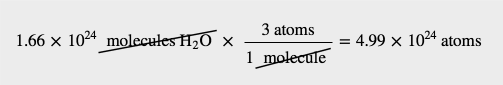Test Yourself

How many molecules are present in 4.61 × 10−2 mol of O2?

Answer

2.78 × 1022 molecules

How big is a mole? It is very large. Suppose you had a mole of dollar bills that need to be counted. If everyone on earth (about 6 billion people) counted one bill per second, it would take about 3.2 million years to count all the bills. A mole of sand would fill a cube about 32 km on a side. A mole of pennies stacked on top of each other would have about the same diameter as our galaxy, the Milky Way. A mole is a lot of things—but atoms and molecules are very tiny. One mole of carbon atoms would make a cube that is 1.74 cm on a side, small enough to carry in your pocket.

Why is the mole unit so important? It represents the link between the microscopic and the macroscopic, especially in terms of mass. A mole of a substance has the same mass in grams as one unit (atom or molecules) has in atomic mass units. The mole unit allows us to express amounts of atoms and molecules in visible amounts that we can understand.

For example, we already know that, by definition, a mole of carbon has a mass of exactly 12 g. This means that exactly 12 g of C has 6.022 × 1023 atoms:

12 g C = 6.022 × 1023 atoms C

We can use this equality as a conversion factor between the number of atoms of carbon and the number of grams of carbon. How many grams are there, say, in 1.50 × 1025 atoms of carbon? This is a one-step conversion: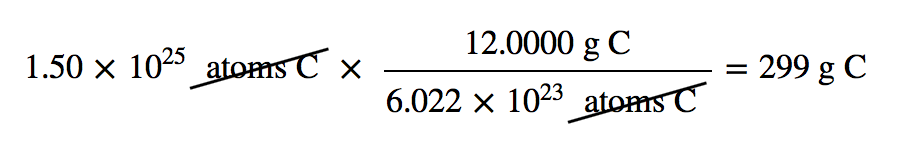But it also goes beyond carbon. Previously we defined atomic and molecular masses as the number of atomic mass units per atom or molecule. Now we can do so in terms of grams. The atomic mass of an element is the number of grams in 1 mol of atoms of that element, while the molecular mass of a compound is the number of grams in 1 mol of molecules of that compound. Sometimes these masses are called molar masses to emphasize the fact that they are the mass for 1 mol of things. (The term molar is the adjective form of mole and has nothing to do with teeth.)

Here are some examples. The mass of a hydrogen atom is 1.0079 u; the mass of 1 mol of hydrogen atoms is 1.0079 g. Elemental hydrogen exists as a diatomic molecule, H2. One molecule has a mass of 1.0079 + 1.0079 = 2.0158 u, while 1 mol H2 has a mass of 2.0158 g. A molecule of H2O has a mass of about 18.01 u; 1 mol H2O has a mass of 18.01 g. A single unit of NaCl has a mass of 58.45 u; NaCl has a molar mass of 58.45 g. In each of these moles of substances, there are 6.022 × 1023 units: 6.022 × 1023 atoms of H, 6.022 × 1023 molecules of H2 and H2O, 6.022 × 1023 units of NaCl ions. These relationships give us plenty of opportunities to construct conversion factors for simple calculations.

### Example 4

What is the molar mass of C6H12O6?

Solution

To determine the molar mass, we simply add the atomic masses of the atoms in the molecular formula but express the total in grams per mole, not atomic mass units. The masses of the atoms can be taken from the periodic table or the list of elements in Chapter 17 “Appendix: Periodic Table of the Elements”:

 6 C = 6 × 12.011 = 72.066 12 H = 12 × 1.0079 = 12.0948 6 O = 6 × 15.999 = 95.994 TOTAL = 180.155 g/mol

Per convention, the unit grams per mole is written as a fraction.

Test Yourself

What is the molar mass of AgNO3?

Answer

169.87 g/mol

Knowing the molar mass of a substance, we can calculate the number of moles in a certain mass of a substance and vice versa, as these examples illustrate. The molar mass is used as the conversion factor.

### Example 5

What is the mass of 3.56 mol of HgCl2? The molar mass of HgCl2 is 271.49 g/mol.

Solution

Use the molar mass as a conversion factor between moles and grams. Because we want to cancel the mole unit and introduce the gram unit, we can use the molar mass as given: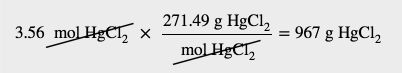Test Yourself

What is the mass of 33.7 mol of H2O?

Answer

607 g

### Example 6

How many moles of H2O are present in 240.0 g of water (about the mass of a cup of water)?

Solution

Use the molar mass of H2O as a conversion factor from mass to moles. The molar mass of water is (1.0079 + 1.0079 + 15.999) = 18.015 g/mol. However, because we want to cancel the gram unit and introduce moles, we need to take the reciprocal of this quantity, or 1 mol/18.015 g: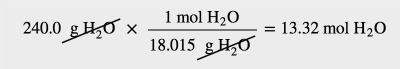Test Yourself

How many moles are present in 35.6 g of H2SO4 (molar mass = 98.08 g/mol)?

Answer

0.363 mol

Other conversion factors can be combined with the definition of mole—density, for example.

### Example 7

The density of ethanol is 0.789 g/mL. How many moles are in 100.0 mL of ethanol? The molar mass of ethanol is 46.08 g/mol.

Solution

Here, we use density to convert from volume to mass and then use the molar mass to determine the number of moles.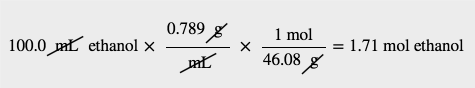Test Yourself

If the density of benzene, C6H6, is 0.879 g/mL, how many moles are present in 17.9 mL of benzene?

Answer

0.201 mol

### Key Takeaways

• The mole is a key unit in chemistry.
• The molar mass of a substance, in grams, is numerically equal to one atom’s or molecule’s mass in atomic mass units.

### Exercises

1. How many atoms are present in 4.55 mol of Fe?

2. How many atoms are present in 0.0665 mol of K?

3. How many molecules are present in 2.509 mol of H2S?

4. How many molecules are present in 0.336 mol of acetylene (C2H2)?

5. How many moles are present in 3.55 × 1024 Pb atoms?

6. How many moles are present in 2.09 × 1022 Ti atoms?

7. How many moles are present in 1.00 × 1023 PF3 molecules?

8. How many moles are present in 5.52 × 1025 penicillin molecules?

9. Determine the molar mass of each substance.

a)  Si

b)  SiH4

c)  K2O

10.  Determine the molar mass of each substance.

a)  Cl2

b)  SeCl2

c)  Ca(C2H3O2)2

11.  Determine the molar mass of each substance.

a)  Al

b)  Al2O3

c)  CoCl3

12.  Determine the molar mass of each substance.

a)  O3

b)  NaI

c)  C12H22O11

13.  What is the mass of 4.44 mol of Rb?

14.  What is the mass of 0.311 mol of Xe?

15.  What is the mass of 12.34 mol of Al2(SO4)3?

16.  What is the mass of 0.0656 mol of PbCl2?

17.  How many moles are present in 45.6 g of CO?

18.  How many moles are present in 0.00339 g of LiF?

19.  How many moles are present in 1.223 g of SF6?

20.  How many moles are present in 48.8 g of BaCO3?

21.  How many moles are present in 54.8 mL of mercury if the density of mercury is 13.6 g/mL?

22.  How many moles are present in 56.83 mL of O2 if the density of O2 is 0.00133 g/mL?

Answers

1.

2.74 × 1024 atoms
3.  1.511 × 1024 molecules
5.  90 mol

7.
0.166 mol

9.

a)  28.086 g

b)  32.118 g

c)  94.195 g

11.

a)  26.981 g

b)  101.959 g

c)  165.292 g

13.  379 g
15.  4,222 g
17.  1.63 mol
19.  0.008374 mol
21.  3.72 mol

## LicenseThe Mole by Jessie A. Key is licensed under a Creative Commons Attribution-NonCommercial-ShareAlike 4.0 International License, except where otherwise noted.